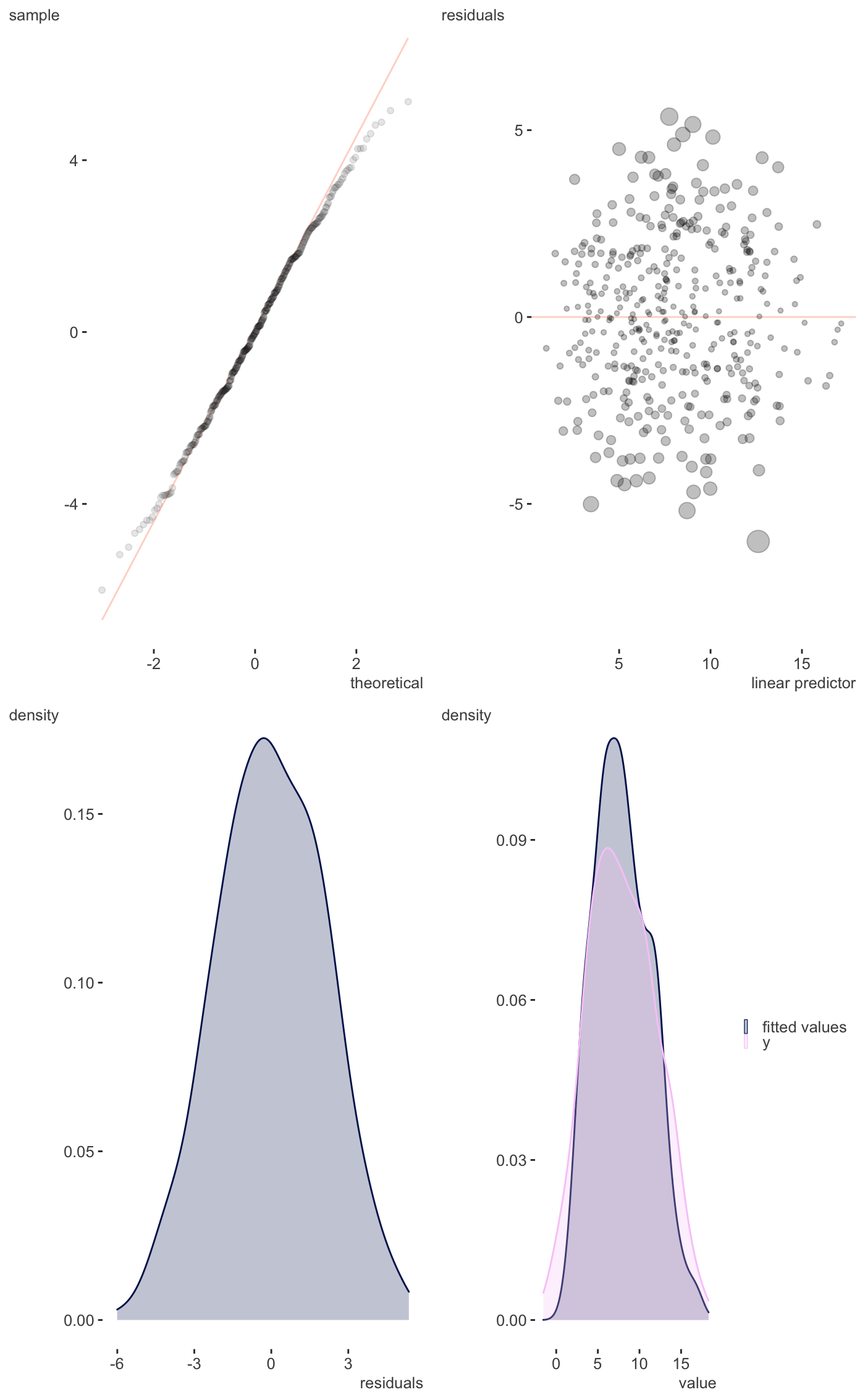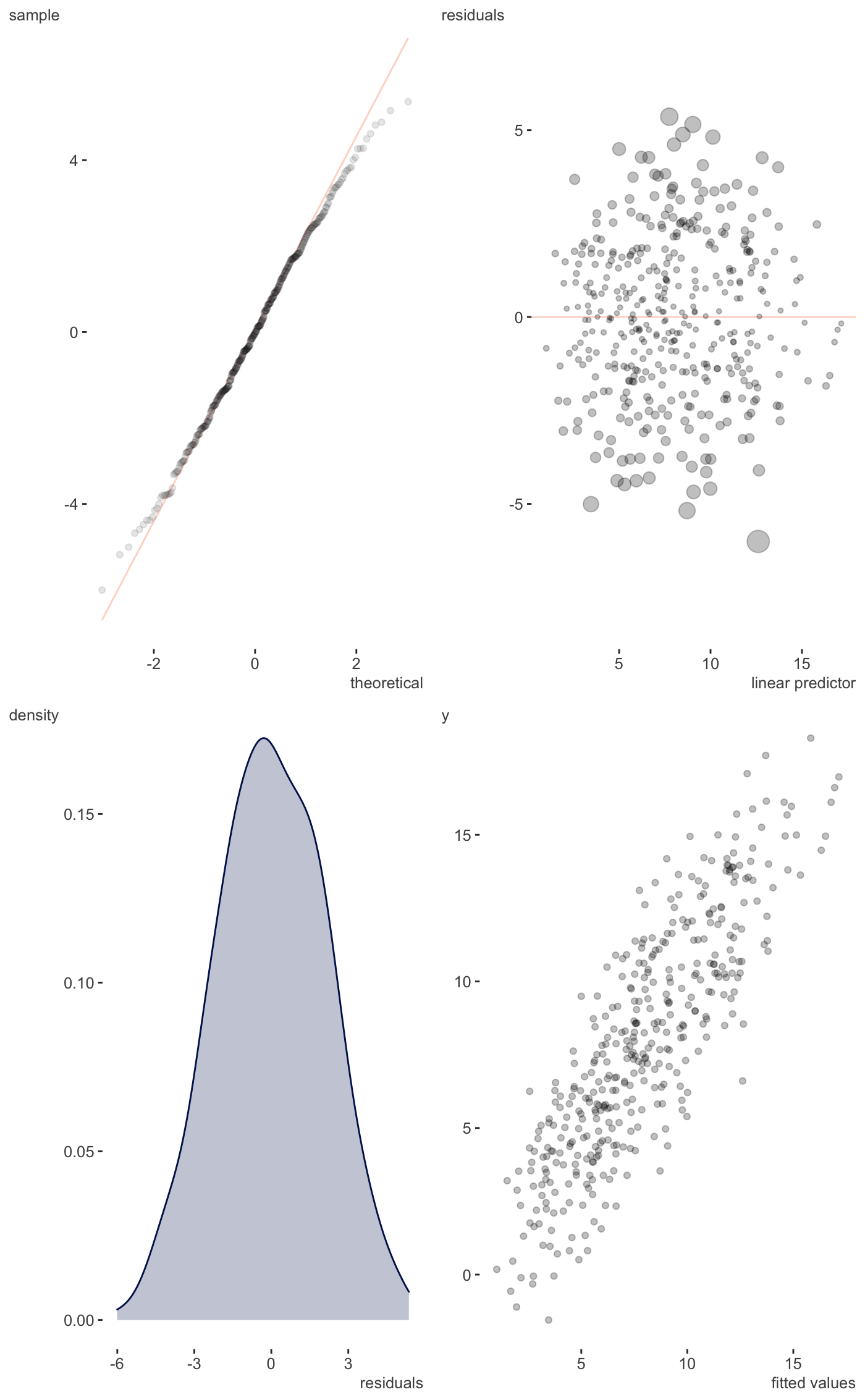Residual plots etc.

plot_gam_check(
model,
single_page = TRUE,
type = "deviance",
scatter = FALSE,
kcheck = FALSE
)

## Arguments

model The mgcv gam model Plot all on a single page. Requires grid.arrange The type of residuals wanted. Usually one of "deviance", "pearson","scaled.pearson", "working", or "response"., See residuals.gam. Whether to plot fitted vs. observed target variable as scatter plot or density. Default is FALSE (density). If you want the slightly less verbose basis dimension (k) checking results. Default is FALSE. When true, only uses the defaults of gam.check. If you need to do more, use mgcv.

## Value

A ggplot that provides visual inspection of residuals and more.

## Details

Just a single page version of gam.check.

gam.check

Other model visualization: plot_coefficients.brmsfit(), plot_coefficients.lm(), plot_coefficients.merMod(), plot_coefficients(), plot_gam_2d(), plot_gam_3d(), plot_gam()

## Examples

library(mgcv); library(visibly)

d <- gamSim(1, n = 400)
#> Gu & Wahba 4 term additive model
g_fit <- gam(y ~ s(x0) + s(x1) + s(x2) + s(x3), data = d)

plot_gam_check(g_fit)plot_gam_check(g_fit, scatter=TRUE)